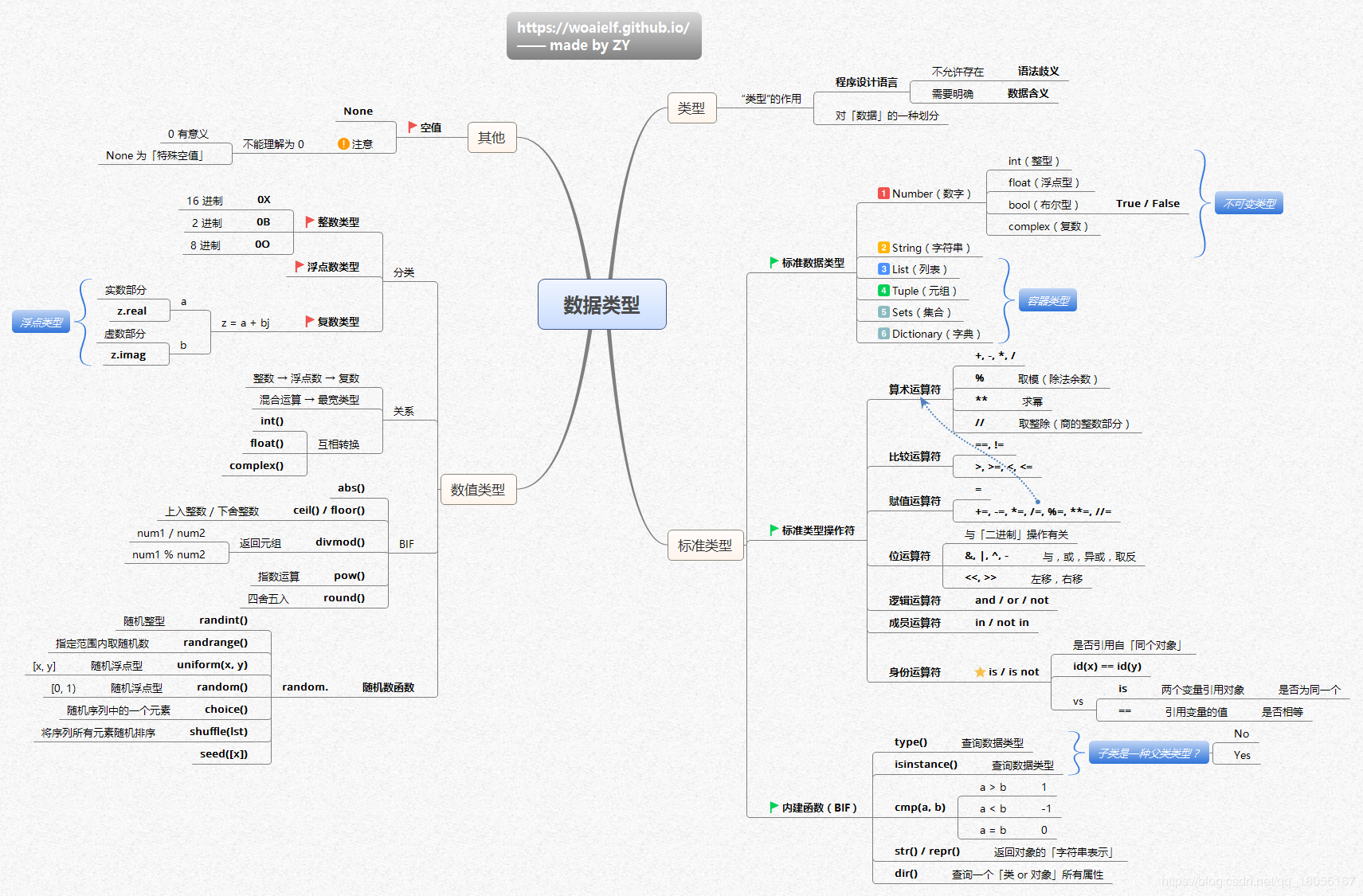#XxwGit 的个人博客

Python的基本数据类型
/

## Python的基本数据类型### Number：包括整数和浮点数

Python3 支持 int、float、bool、complex（复数）。

``````>>> a, b, c, d = 20, 5.5, True, 4+3j
>>> print(type(a), type(b), type(c), type(d))
<class 'int'> <class 'float'> <class 'bool'> <class 'complex'>
``````
``````print(type(1))
#输出<class 'int'>
print(type(1.1111111111))
#输出<class 'float'>
print(type(1+1.1))
#输出<class 'float'>
print(type(1+1.0))
#输出<class 'float'>
print(type(1+1))
#输出<class 'int'>
print(type(1*1.0))
#输出<class 'float'>
print(type(1+1))
#输出<class 'int'>
print(type(2/2))
#输出<class 'float'>
print(type(2//2))
#输出<class 'int'>
``````

10进制：0，1，2，3，4，5，。。。。，9，10，11.。。。
8进制：0，1，2，3，。。。。，7，10，11，12.。。。，17，20.。。。
2进制：0，1，10，11，100.。。。

``````#二进制数字在python中表示需要在前面加上0b
print(0b10)
#输出 2
#八进制数字在Python中表示需要在前面加上0o
print(0o10)
#输出 8
#十六进制数字在Python中表示需要在前面加上0x
print(0x1f)
#输出 31

#进制转换
#将其他进制转换为二进制，使用bin方法
print(bin(10))
#输出 0b1010
print(bin(0xE))
#输出 0b1110
print(bin(0o11))
#输出 0b1001
#将其他进制转换为十进制，使用int()方法
#将其他进制转换为十六进制，使用hex()方法
#将其他进制转换为十=八进制，使用oct(方法)
``````
``````print(type(True))
print(type(False))
print(int(True))
print(int(False))
print(bool(1))
print(bool(0))
#输出<class 'bool'>
#输出<class 'bool'>
#输出1
#输出0
#输出True
#输出False
#只有数字0，None和空值可以表示false，其余所有的例如数组整数小数等等都代表True
``````

1. Python可以同时为多个变量赋值，如a, b = 1, 2。
2. 一个变量可以通过赋值指向不同类型的对象。
3. 数值的除法包含两个运算符：/ 返回一个浮点数，// 返回一个整数。
4. 在混合计算时，Python会把整型转换成为浮点数。

### Str：字符串

Python中的字符串用单引号 ' 或双引号 " 括起来，同时使用反斜杠 \ 转义特殊字符。``````#!/usr/bin/python3

str = 'Runoob'

print (str)          # 输出字符串
print (str[0:-1])    # 输出第一个到倒数第二个的所有字符
print (str)       # 输出字符串第一个字符
print (str[2:5])     # 输出从第三个开始到第五个的字符
print (str[2:])      # 输出从第三个开始的后的所有字符
print (str * 2)      # 输出字符串两次
print (str + "TEST") # 连接字符串
``````

Runoob
Runoo
R
noo
noob
RunoobRunoob
RunoobTEST

Python 使用反斜杠()转义特殊字符，如果你不想让反斜杠发生转义，可以在字符串前面添加一个 r，表示原始字符串：

``````>>> print('Ru\noob')
Ru
oob
>>> print(r'Ru\noob')
Ru\noob
>>>
``````

``````>>>word = 'Python'
>>> print(word, word)
P n
>>> print(word[-1], word[-6])
n P
``````

1. 反斜杠可以用来转义，使用r可以让反斜杠不发生转义。
2. 字符串可以用+运算符连接在一起，用*运算符重复。
3. Python中的字符串有两种索引方式，从左往右以0开始，从右往左以-1开始。
4. Python中的字符串不能改变。

### List：列表

List（列表） 是 Python 中使用最频繁的数据类型。``````#!/usr/bin/python3

list = [ 'abcd', 786 , 2.23, 'runoob', 70.2 ]
tinylist = [123, 'runoob']

print (list)            # 输出完整列表
print (list)         # 输出列表第一个元素
print (list[1:3])       # 从第二个开始输出到第三个元素
print (list[2:])        # 输出从第三个元素开始的所有元素
print (tinylist * 2)    # 输出两次列表
print (list + tinylist) # 连接列表
``````

['abcd', 786, 2.23, 'runoob', 70.2]
abcd
[786, 2.23]
[2.23, 'runoob', 70.2]
[123, 'runoob', 123, 'runoob']
['abcd', 786, 2.23, 'runoob', 70.2, 123, 'runoob']

``````>>>a = [1, 2, 3, 4, 5, 6]
>>> a = 9
>>> a[2:5] = [13, 14, 15]
>>> a
[9, 2, 13, 14, 15, 6]
>>> a[2:5] = []   # 将对应的元素值设置为 []
>>> a
[9, 2, 6]
``````

### Tuple：元组

``````#!/usr/bin/python3

tuple = ( 'abcd', 786 , 2.23, 'runoob', 70.2  )
tinytuple = (123, 'runoob')

print (tuple)             # 输出完整元组
print (tuple)          # 输出元组的第一个元素
print (tuple[1:3])        # 输出从第二个元素开始到第三个元素
print (tuple[2:])         # 输出从第三个元素开始的所有元素
print (tinytuple * 2)     # 输出两次元组
print (tuple + tinytuple) # 连接元组
``````

('abcd', 786, 2.23, 'runoob', 70.2)
abcd
(786, 2.23)
(2.23, 'runoob', 70.2)
(123, 'runoob', 123, 'runoob')
('abcd', 786, 2.23, 'runoob', 70.2, 123, 'runoob')

``````>>>tup = (1, 2, 3, 4, 5, 6)
>>> print(tup)
1
>>> print(tup[1:5])
(2, 3, 4, 5)
>>> tup = 11  # 修改元组元素的操作是非法的
Traceback (most recent call last):
File "<stdin>", line 1, in <module>
TypeError: 'tuple' object does not support item assignment
``````

tup1 = () # 空元组
tup2 = (20,) # 一个元素，需要在元素后添加逗号

string、list 和 tuple 都属于 sequence（序列）。

1. 与字符串一样，元组的元素不能修改。
2. 元组也可以被索引和切片，方法一样。
3. 注意构造包含 0 或 1 个元素的元组的特殊语法规则。
4. 元组也可以使用+操作符进行拼接。

### Set：集合

parame = {value01,value02,...}

set(value)

``````#!/usr/bin/python3

student = {'Tom', 'Jim', 'Mary', 'Tom', 'Jack', 'Rose'}

print(student)   # 输出集合，重复的元素被自动去掉

# 成员测试
if 'Rose' in student :
print('Rose 在集合中')
else :
print('Rose 不在集合中')

# set可以进行集合运算
b = set('alacazam')

print(a)

print(a - b)     # a 和 b 的差集

print(a | b)     # a 和 b 的并集

print(a & b)     # a 和 b 的交集

print(a ^ b)     # a 和 b 中不同时存在的元素
``````

{'Mary', 'Jim', 'Rose', 'Jack', 'Tom'}
Rose 在集合中
{'b', 'a', 'c', 'r', 'd'}
{'b', 'd', 'r'}
{'l', 'r', 'a', 'c', 'z', 'm', 'b', 'd'}
{'a', 'c'}
{'l', 'r', 'z', 'm', 'b', 'd'}

### Dictionary：字典

``````#!/usr/bin/python3

dict = {}
dict['one'] = "1 - 菜鸟教程"
dict     = "2 - 菜鸟工具"

tinydict = {'name': 'runoob','code':1, 'site': 'www.runoob.com'}

print (dict['one'])       # 输出键为 'one' 的值
print (dict)           # 输出键为 2 的值
print (tinydict)          # 输出完整的字典
print (tinydict.keys())   # 输出所有键
print (tinydict.values()) # 输出所有值
``````

1 - 菜鸟教程
2 - 菜鸟工具
{'name': 'runoob', 'code': 1, 'site': 'www.runoob.com'}
dict_keys(['name', 'code', 'site'])
dict_values(['runoob', 1, 'www.runoob.com'])

``````>>>dict([('Runoob', 1), ('Google', 2), ('Taobao', 3)])
{'Taobao': 3, 'Runoob': 1, 'Google': 2}

>>> {x: x**2 for x in (2, 4, 6)}
{2: 4, 4: 16, 6: 36}

{'Runoob': 1, 'Google': 2, 'Taobao': 3}
``````

1. 字典是一种映射类型，它的元素是键值对。
2. 字典的关键字必须为不可变类型，且不能重复。
3. 创建空字典使用 { }。

### Python数据类型转换

int(x [,base])将x转换为一个整数
float(x)将x转换到一个浮点数
complex(real [,imag])创建一个复数
str(x)将对象 x 转换为字符串
repr(x)将对象 x 转换为表达式字符串
eval(str)用来计算在字符串中的有效Python表达式,并返回一个对象
tuple(s)将序列 s 转换为一个元组
list(s)将序列 s 转换为一个列表
set(s)转换为可变集合
dict(d)创建一个字典。d 必须是一个 (key, value)元组序列。
frozenset(s)转换为不可变集合
chr(x)将一个整数转换为一个字符
ord(x)将一个字符转换为它的整数值
hex(x)将一个整数转换为一个十六进制字符串
oct(x)将一个整数转换为一个八进制字符串

-----------------------------------------本章完-----------------------------------------